# MATHEMATICS — 2004 (Set III — Compartment Delhi)

Except for the following questions, all the remaining questions have been asked in Set I and Set II .

Q.2. If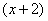is a factor of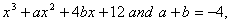find the values of a and b.

Q. 5. A loan has to be paid back in two equal annual instalments. If the rate of interest is 15% per annum, compounded annually, and each instalment is Rs. 2645, find the loan and total interest charged.

Q. 6. A computer is available for Rs. 39,000 cash or Rs. 17,000 as cash down payment followed by 5 monthly instalments of Rs. 4,800 each. Find the rate of interest under the instalment plan.

Q. 11. A two-digit number is 5 times the sum of its digits and is also equal to 5 more than twice the product of its digits. Find the number.

Q. 17. A card is drawn at random from a pack of 52 playing cards. Find the probability that the card drawn is neither a red card nor a black king.

Q. 20. The coordinates of the mid-point of the line joining the points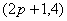and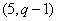are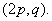find the values of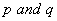.

Q. 24. The annual income of Sameer is Rs. 3,80,000 (exclusive of HRA). He contributes Rs. 6,000 per month towards his provident fund and pays an annual premium of Rs. 18,000 for his LIC policy. If he pays Rs. 6,000 per month towards income tax for the first eleven months of the year, find his income tax liability for the last month of the year.

for calculating income tax, use the following:

 (a) Standard Deduction Gross income Deduction (i) Upto Rs.75,000 (ii) From Rs.75,001 to Rs.5,00,000 (iii) More than Rs,5,00,000 40% of gross income Rs.30,000 Rs.20,000 (b) Rates of Taxes Slab Income tax (i) Upto Rs. 50,000 (ii) From Rs. 50,001 to Rs. 60,000 (iii) From Rs. 60,001 to Rs. 1,50,000 (iv) Rs. 1.50,000 and above No tax 10% of the amount exceeding Rs.50,000 Rs.1,000+20% of the amount exceeding Rs.60,000 Rs.19,000+30% of the amount exceeding Rs.1,50,000 (c) Rebate in Tax (i) 20% of the savings subject to a maximum of Rs. 14,000 if the gross income is upto Rs. 1,50,000. (ii)15% of the savings subject to a maximum of Rs. 10,500 if the gross income is from Rs. 1,50,001 to Rs. 5,00,000.
Maths 2004 Question Papers Class X
CBSE 2004 Question Papers Class X